Announcements

# Power Management ICs Forum Discussions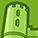Level 3
Level 3# LLC Design Frequency Range Problem

Hello together,

I'm a bit confused. So I have drawn the gain-curves of the LLC converter. And they look fine on the nominal frequency scale: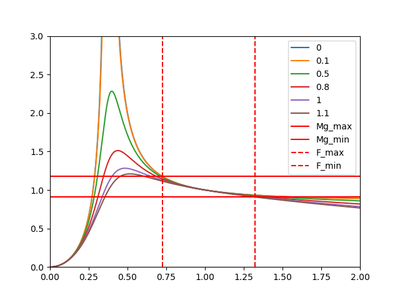In the diagram the numbers in the legend are the amount of load with 1 being 1500W at 100V output load. The formula used are:

Mg = 1/sqrt(Qe**2*(-fn + 1/fn)**2 + (1 + 1/Ln - 1/(Ln*fn**2))**2)
with fn=fsw/fr; Ln=Lm/Lr; Qe=sqrt(Lr/Cr)/Rac; Rac=8*Vout**2*n**2/(pi**2*Pout)

But when i determine the resonant frequencies for different Loads (graphically determined - cross checked with calculation) and draw the gain-curves across the switching frequency the area of the frequency range is way off than the desired 500kHz resonant. It's some where between 169819Hz -298807Hz.

The NullMg represents the gain-curve for the Null Load case.
The MaxiMg represents the gain-curve for the Maximal Load case, which is here the 1500W.
The FullMg represents the gain-curve for the Full Load case.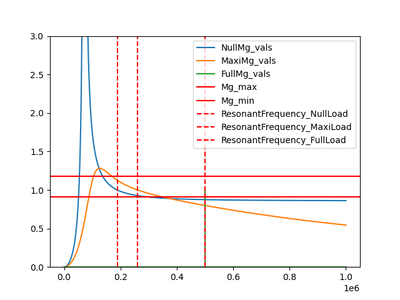I was expecting a wider range when plotting the gain-curves with the  resonant frequency for each load. Due to the relation between the resonant frequency and the load. But I did not expect such a big deviation.

Is this big deviation normal, or is maybe something with my fundamentals flawed?

PS: I used Ln=6; Qe=0.4 to determine the resonant circuit.

It should be noted that I know that choosing a smaller Ln results in a narrower frequency range, but additionally it results in increased conduction losses and decreased commutation losses.
Additionally a big Ln results in a big Lm, which is needed to keep the ZVS.

1 SolutionModeratorModerator# Re: LLC Design Frequency Range Problem

Hi,

Q: But I still don't quite get why only the resonant frequency at full-load is being used. And not the other resonant frequencies. I've read that the resonant frequency at full load is always applicable no matter the load condition. While the resonant frequency at no-load is only true at no-load

1. At the resonant frequency, the converter will perform the highest efficiency due to
ZCS Off at Rectifier diodes
ZVS ON at Primary switches
The resonant tank has unity gain and the best-optimized operation

2. At below Resonant frequency circulating currents causes excess energy loss at the primary side.

3. At the above Resonant frequency, ZCS losses are unavoidable in rectifier diodes.

Since the resonant frequency provides optimal performance, based on the end application the designer can choose to operate the converter at/around the resonant frequency to get the maximum benefits of the resonant converter

Thanks,

SPCH

6 RepliesModeratorModerator# Re: LLC Design Frequency Range Problem

Hi,

Thanks for posting at Infineon Community.

As per your data, we understand that the Gain curves were drawn between,

1. Normalized Frequency (Fn) Vs Gain (Mg) in 1st Fig.
2. Switching Frequency (Fsw) Vs Gain (Mg) in 2nd Fig.

The only difference between the gain plots is Normalized frequency and switching frequency on X-Axis. So, we are recommending you cross-check The gain equation while arriving with 2nd Fig. Where normalized frequency (Fn) should be replaced with Switching frequency (Fsw).

If you have chosen Resonant tank parameters (Lr and Cr) with 500kHz Resonant frequency in your design, it should reflect the unity gain at that frequency in the Gain Curves. And also switching frequency sweep should be around the Resonant frequency to maintain the regulation, it can’t be 169819Hz -298807Hz.

However, there can be a small difference in frequency in the order of 5kHz when compared with theory and practical since we are using FHA-based gain curves.

Thanks,

SPCH.Level 3
Level 3# Re: LLC Design Frequency Range Problem

Hi,

In the second graph the i have used the resonant frequency for each load. Due to the fact, that the resonant frequency is load dependent.

When using the Full-Load resonant frequency with 500kHz for each load, in order to calculate the fn used for the gain equation, the graph looks similar to the first one I've posted.

But due to the smaller resonant frequency for No-load I used the calculated No-Load resonant frequency for the Qe=0 case in order to determine the real minimum necessary switching frequency to get to Mg_max. For the maximum load case I determined the resonant frequency for the desired load as well and with it I determined the fn, which I used to calculate the gain curve.

The normalized frequency Fn=Fsw/Fr depends on the switching frequency and the resonant frequency.ModeratorModerator# Re: LLC Design Frequency Range Problem

Hi,

Thanks for posting at Infineon Community.

At no-load the frequency can't be 223KHz it should be above the resonant frequency. Please check.
@ no-load converter won't operate at a lower resonant frequency.

As per your data from 1st post & 1st Fig. Below are the approximated values.

 FR 500 KHz FSW-MAX. 650 KHz At No-load

FN approximated as 1.3 Refer Below Fig.
FR=500KHz
By using FN=FSW/FR formula FSW-MAX can be calculated, Refer above table for the value.

To match both the gain curves we recommend you to use

You can follow same procedure to estimate full load operating frequency also.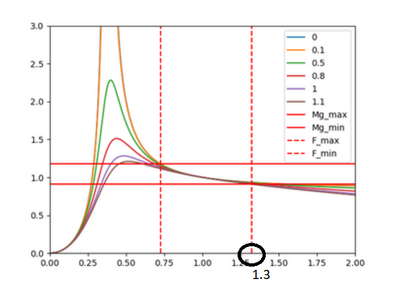Below are the LLC Design Guide links you can refer to for further understanding.

https://www.infineon.com/dgdl/Application_Note_Resonant+LLC+Converter+Operation+and+Design_Infineon....

https://www.infineon.com/cms/en/product/evaluation-boards/eval-600w-12v-llc-a/

Thanks,

SPCHLevel 3
Level 3# Re: LLC Design Frequency Range Problem

Hi,

I understand how you get the f_max at no-load and the f_min at full-load. But I am still a bit confused about the resonant frequency. Due to the fact, that the resonant frequency seems to be related to the load. Which can be seen in the following picture: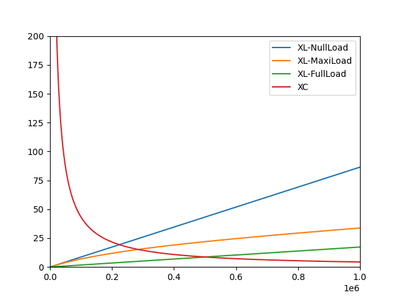The intersection of the XC and the XL lines are the resonant frequencies.
XC=XLr+XLm||Rac

It is obvious to me that operation at, below and above resonant frequency offers different advantages and drawbacks. The LLC is most of the time designed to operate in the vicinity of the f_0 (resonant frequency at full-load).

But I still don't quite get why only  the resonant frequency at full-load is being used. And not the other resonant frequencies. I've read that the resonant frequency at full-load is always applicable no matter the load condition. While the resonant frequency at no-load is only true at no-load.

Can one maybe explain to me, why the different resonant frequencies for different load conditions can be ignored?

Best regardsLevel 3
Level 3# Re: LLC Design Frequency Range Problem

Hi,

I think I got it now. Each gain-curve has two resonant-peaks one is load dependent (fp=1/(2*pi*sqrt(Cr*(Lr+Lm)))) and the other is not (fr=1/(2*pi*sqrt(Cr*Lr))). The peaks of the gain-curves (which are dependent on the load) are between those two resonant frequencies.
Therefor the normalized frequency must be with respect to fr, where the gain for all load conditions is unity.

Thanks for clarifying this.ModeratorModerator# Re: LLC Design Frequency Range Problem

Hi,

Q: But I still don't quite get why only the resonant frequency at full-load is being used. And not the other resonant frequencies. I've read that the resonant frequency at full load is always applicable no matter the load condition. While the resonant frequency at no-load is only true at no-load

1. At the resonant frequency, the converter will perform the highest efficiency due to
ZCS Off at Rectifier diodes
ZVS ON at Primary switches
The resonant tank has unity gain and the best-optimized operation

2. At below Resonant frequency circulating currents causes excess energy loss at the primary side.

3. At the above Resonant frequency, ZCS losses are unavoidable in rectifier diodes.

Since the resonant frequency provides optimal performance, based on the end application the designer can choose to operate the converter at/around the resonant frequency to get the maximum benefits of the resonant converter

Thanks,

SPCH